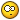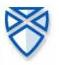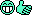# Problem with equations like ax^2+bx+c = 0

9 replies to this topic

### #1Chapas

Chapas

Newbie

•• Members
•• 19 posts

• Calculators:
Casio 9750, CP300

Posted 28 June 2004 - 03:20 PM

How can i resolve a equation like : ax^2+bx+c = 0 , in my cp300 ??

I put some equantion in solve, and some results are correct others are wrong !!Could anybody explain to me what is going wrong ?

### #2Overlord

Overlord

Casio Technician

•• Moderator
•• 355 posts
• Gender:Male
• Location:Brussels - Belgium
• Interests:Math Researcher

• Calculators:
My head - C300 OS 3.00 - G100 Rom 1.02 - G65 - G60 - G25

Posted 28 June 2004 - 03:42 PM

can you give an example of equation and results ?

check also that your variables haven't wrong values in memory..

### #3SoftCalc

SoftCalc

Casio Technician

•• Members
•• 406 posts
• Location:Portland, OR USA

• Calculators:
ClassPad 300 , AFX 2.0, HP-48/49/50, TI-89/92/Voyager, HP Expander, etc...

Posted 28 June 2004 - 05:37 PM

Try clearing all variables first by entering Clear_a_z. If this doesn't work give some examples...

### #4Chapas

Chapas

Newbie

•• Members
•• 19 posts

• Calculators:
Casio 9750, CP300

Posted 28 June 2004 - 07:07 PM

Another question

When we put in SOLVE MENU the equation x^2-4 = 0 , the result should be x= -2 and x=2 , but in result oly appears x=2 , WHY??

### #5Daruosh

Daruosh

Casio Freak

•• Members
•• 285 posts
• Gender:Male
• Location:Tehran - Iran
• Interests:Computer Programming, Electronics, Image Processing, Neural Networks, AI, System Development, Calc, Guitar, Music,

• Calculators:
CP 300 OS 3.03, Algebra FX 2.0 Plus ROM 1.05, CG-20

Posted 28 June 2004 - 07:56 PM

When we put in SOLVE MENU the equation x^2-4 = 0 , the result should be x= -2 and x=2 , but in result oly appears x=2 , WHY??

I get {x=-2,x=2}

try solve(x^2-4=0)

### #6Chapas

Chapas

Newbie

•• Members
•• 19 posts

• Calculators:
Casio 9750, CP300

Posted 28 June 2004 - 08:06 PM

In Main Menu i appers {x=-2, x=2} !!

But why don't appers the same in the solve menu ?

### #7SoftCalc

SoftCalc

Casio Technician

•• Members
•• 406 posts
• Location:Portland, OR USA

• Calculators:
ClassPad 300 , AFX 2.0, HP-48/49/50, TI-89/92/Voyager, HP Expander, etc...

Posted 28 June 2004 - 08:24 PM

In Main Menu i appers {x=-2, x=2} !!
But why don't appers the same in the solve menu ?

What do you mean by "solve menu"? Do you mean the NumSolve application? If so, the NumSolve application solves numerically, not symbolically. You won't get all roots. There is't even a guarantee you'll get an exact solution.

In the NumSolve application you have to give an initial guess (x=) and it will attempt to find the closest solution. Enter x=1 and it will find the solution x=2. Enter x=-1 and it will find the solution x=-2.

If you want a complete, symbolic solution you should try using "solve(" in MAIN first.

### #8Overlord

Overlord

Casio Technician

•• Moderator
•• 355 posts
• Gender:Male
• Location:Brussels - Belgium
• Interests:Math Researcher

• Calculators:
My head - C300 OS 3.00 - G100 Rom 1.02 - G65 - G60 - G25

Posted 28 June 2004 - 08:43 PM

i think the numeric solver uses a newton raphson method (or a variant like the secant method) that tries to find the nearst root from the begin point (numerically so not the exact value).

=>
1. it can found only 1 value at one time
2. you can't be sure it will find a solution even if there are solutions (for a success with n-r you must satisfy a mathematical condition ; most time it will be satisfied if your guess is relatively "close" to the solution or if the function is "regular" enough
3. sometimes it can give a erratic solution... don't forget to check the solution

but, it can estimate solutions that the calc or even we can't find by symbolic methods(and it can also be useful for teachers to test the students at an numeric methods exam :-/ grrrrr...)

### #9R00KIE

R00KIE

Casio Freak

•• Members
•• 155 posts
• Location:Portugal
• Interests:Electronics, games, programming

• Calculators:
HP49G ROM 1.24; CASIO CFX-9850GB PLUS;CASIO FX-6300G; CASIO FX-82TL

Posted 02 July 2004 - 11:24 AM

But don't underestimate the power of the numeric solvers because when you can't solve it in exact/symbolic mode you need to turn to numeric mode.

### #10SoftCalc

SoftCalc

Casio Technician

•• Members
•• 406 posts
• Location:Portland, OR USA

• Calculators:
ClassPad 300 , AFX 2.0, HP-48/49/50, TI-89/92/Voyager, HP Expander, etc...

Posted 02 July 2004 - 04:51 PM

I totally agree. I calculator wouldn't be a calculator if it didn't have a good numeric solver, and Casio's root solver does an excellent job.I just wanted to make the point that a numeric solver isn't guaranteed to get a correct solution. People often expect that a calculator must always give the correct answer or it is some type of bug. A numeric root solver is just riding the curve trying to find the answer.

#### 0 user(s) are reading this topic

0 members, 0 guests, 0 anonymous users